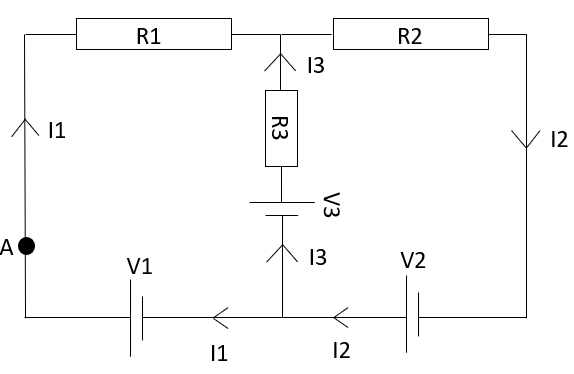# 2.4.4 Worked example 2.3

Course subject(s) Module 2: Electricity & magnetism

For this worked example we will look at the electrical circuit below.In this circuit, R1=20 Ω , R2=10 Ω , R3=5.0 Ω, V1=10 V, V2=20 V and V3=30 V

Note: the numerical answers obtained in the video are incorrect. The derivation however is correct.